Contents Articles Behaviors Books Director News Director Web Sites FAQ Games Mailing Lists News Groups Project Examples Reviews Software Tools Useful Web Sites Utilities Xtras

 Don't miss these#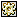RGB to HSV, HSV to RGB

 Compatibilities: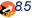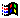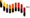This item has not yet been rated

Author: Andrew Morton

Converts rgb values to hsv and back.

 -- RGB<->HSV conversion -- RGB, each 0 to 255 -- H = 0.0 to 360.0 (corresponding to 0..360.0 degrees around hexcone) -- S = 0.0 (shade of gray) to 1.0 (pure color) -- V = 0.0 (black) to 1.0 (white) -- Based on C Code in "Computer Graphics -- Principles and Practice," -- Foley et al, 1996, pp. 592,593. -- Converted to Lingo by Andrew Morton akm@in-press.co.uk on RGBToHSV(R, G, B)   R=float(R)   G=float(G)   B=float(B)   minVal=min(R, G, B)   V=max(R, G, B)   Delta=V-minVal   -- Calculate saturation: saturation is 0 if r, g and b are all 0   if V=0.0 then     S=0.0   else     S=Delta / V   end if   if S=0.0 then     H=0.0    -- Achromatic: When s = 0, h is undefined but who cares   else       -- Chromatic     if R=V then -- between yellow and magenta [degrees]       H=60.0*(G-B)/Delta     else       if G=V then -- between cyan and yellow         H=120.0+60.0*(B-R)/Delta       else -- between magenta and cyan         H=240.0+60.0*(R-G)/Delta       end if     end if   end if   if H<0.0 then H=H+360.0   -- return a list of values as an rgb object would not be sensible   return [h, s, v/255.0] end RGBtoHSV on HSVtoRGB(h, s, v)   h=float(h)   s=float(s)   v=float(v)   if S=0.0 then   -- color is on black-and-white center line     R=V           -- achromatic: shades of gray     G=V           -- supposedly invalid for h=0 but who cares     B=V   else -- chromatic color     if H=360.0 then  -- 360 degrees same as 0 degrees       hTemp=0.0     else       hTemp=H     end if     hTemp=hTemp/60.0   -- h is now in [0,6)     i=bitOr(hTemp, 0)  -- largest integer <= h     f=hTemp-i          -- fractional part of h     p=V*(1.0-S)     q=V*(1.0-(S*f))     t=V*(1.0-(S*(1.0-f)))     case i of       0:         R = V         G = t         B = p       1:         R = q         G = V         B = p       2:         R = p         G = V         B = t       3:         R = p         G = q         B = V       4:         R = t         G = p         B = V       5:         R = V         G = p         B = q     end case   end if   return rgb(R*255, G*255, B*255) end HSVtoRGB

 Contact MMI 36 South Court Sq Suite 300 Newnan, GA 30263 USA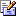Send e-mail